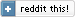(November 2011)

I first met yield and coroutines many years ago, when I was learning Python. I quickly realized that this construct adds both power and elegance to a language; and since then, one of the first things I check when working with a new language, is if it has support for yield.

I am happy to say that most modern languages offer it - but there's a catch: not all of them support it in the same way. This may lead to... unexpected results.

To demonstrate, I will use Python, C# and F#, in implementing the Fibonacci sequence.

## In Python...

```#!/usr/bin/env python

def fib():
a0, a1 = 1, 1
yield a0
yield a1
while a0<1000000:
yield a0+a1
a0, a1 = a1, a0+a1

fibonacciNumbersUpToMillion = fib()
for i in fibonacciNumbersUpToMillion:
print(i)
print(any(i==10946 for i in fibonacciNumbersUpToMillion))
for i in fibonacciNumbersUpToMillion:
print(i)
```
So...
• We initialize a0 and a1 to the first two terms of the sequence
• Yield them back in sequence
• Then do a loop, calculating the next element and yielding it back
Simple, and concise.

But mathematicians will be surprised by the output of this program...

```bash\$ python fib.py
1
1
2
3
5
8
13
...
1346269
2178309
False

bash\$
```
The fibonacciNumbersUpToMillion is a Python generator (since it invokes yield) that indeed returns the Fibonacci sequence, until it passes one million...

But only once!

When we call any to check if 10946 is part of the sequence, we get... False. And the second iteration prints... nothing.

Why?

Because Python has "exhausted" the generator with the print that was done before - the loop has ended, there's no more data returned, StopIteration was raised, and the generator is now empty...

## In C#

```using System;
using System.Collections.Generic;
using System.Linq;
using System.Text;

namespace ConsoleApplication {
class Program {
static IEnumerable<int> fib() {
int a0 = 1;
int a1 = 1;
yield return a0;
yield return a1;
while (a0<1000000) {
yield return a0+a1;
var a2 = a0+a1;
a0 = a1;
a1 = a2;
}
}
static void Main(string[] args) {
var fibonacciNumbersUpToMillion  = fib();
foreach (var x in fibonacciNumbersUpToMillion)
Console.WriteLine(x);
Console.WriteLine(fibonacciNumbersUpToMillion.Contains(10946));
foreach (var x in fibonacciNumbersUpToMillion)
Console.WriteLine(x);
}
}
}
```
As you can see, the code closely follows what the Python code did (albeit with the mandatory namespaces and classes that C# requires). But C# behaves differently:
```bash\$ csc fib.cs
bash\$ ./fib.exe
1
1
2
3
...
1346269
2178309
True
1
1
2
3
...
2178309

bash\$
```
The fibonacciNumbersUpToMillion variable contains a sequence that will *always* carry what one would expect: all the Fibonacci numbers up to a million.

How can C# do it? Why does Python fail to do so?

Because C# paid the price that this demands: When implementing yield, the C# compiler was instructed to perform a code transformation (generating a "magic" class) whenever it meets the construct. This transformation, in plain words, arranges so that the iteration state be kept at the *caller* side, not the *callee* side. In terms of our example above, you can think of iteration state as being managed in the "for" loop that prints - not in the fib() itself, as is done in Python.

If you want to avoid this in Python, make sure you NEVER assign the generator to a variable (in this case, fibonacciNumbersUpToMillion) - because the variable's state will be modified upon use. Instead, use the generator creator every time:

```...

for i in fib():
print(i)

print(any(i==10946 for i in fib()))
```
This way, you "start fresh" every time.

## In F#

```let fib () =
seq {
let mutable a0 = 1
let mutable a1 = 1
yield a0
yield a1
while a0<1000000 do
yield a0+a1
let a2 = a0+a1
a0 <- a1
a1 <- a2
}
```
Ah, now this gets interesting:
```bash\$ fsc.exe fib.fs
yield a1
--------^^^^^^^^

fib.fs(6,9): error FS0407: The mutable variable 'a0' is used in an invalid way.
Mutable variables cannot be captured by closures. Consider eliminating this use of mutation
or using a heap-allocated mutable reference cell via 'ref' and '!'.
```
Listen to what F# says; "mutable variables cannot be captured by closures" - in plain words, the body of fib has *state* inside it, which will be modified upon each iteration over it. And it tells us that we can't do this... at least not with mutable variables.

F# is the descendant of a functional language (OCaml), and it wants its closures to be state-free. In plain words, it wants readers of your code to reason about it in the same way mathematicians do :‑)

But F#, like her mother OCaml (and unlike her crazy aunt Haskell :‑), is practical. She knows that imperative constructs are sometimes the way to go. And it allows you - if you want to - to do the dirty deed and keep state inside:

```let fib () =
seq {
let a0 = ref 1
let a1 = ref 1
yield !a0
yield !a1
while !a0<1000000 do
yield !a0 + !a1
let a2 = !a0 + !a1
a0 := !a1
a1 := a2
}
```
Which works just as C# does (i.e. you can assign this sequence to a variable and re-use it as many times as you want with no problems.

## Conclusion

yield is a very useful language construct, and allows clear and concise code - but be careful of the differences of it's implementation amongst languages.

 Index  CV Updated: Sat Oct 8 12:33:59 2022

The comments on this website require the use of JavaScript. Perhaps your browser isn't JavaScript capable or the script is not being run for another reason. If you're interested in reading the comments or leaving a comment behind please try again with a different browser or from a different connection.